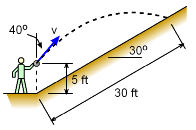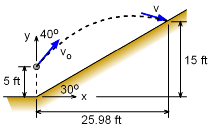Ch 1. Particle General Motion Multimedia Engineering Dynamics Position,Vel & Accel. Accel. varyw/ Time Accel. Constant Rect. Coordinates Norm/Tang. Coordinates Polar Coordinates RelativeMotion
 Chapter - Particle - 1. General Motion 2. Force & Accel. 3. Energy 4. Momentum - Rigid Body - 5. General Motion 6. Force & Accel. 7. Energy 8. Momentum 9. 3-D Motion 10. Vibrations Appendix Basic Math Units Basic Equations Sections Search eBooks Dynamics Fluids Math Mechanics Statics Thermodynamics Author(s): Kurt Gramoll ©Kurt GramollDYNAMICS - EXAMPLEBall Throw Example A small ball is throw up a slope a distance of 30 ft. The slope is 30o from the horizontal and the ball's initial velocity is 40o from the vertical as shown. The ball leaves the persons hand 5 ft from the ground. What is the smallest initial velocity? SolutionVertical and Horizontal Distances The ball travels in both the x and y directions. Two equations will be needed. First, examine the y direction,      y = yo + voyt - 0.5gt2      30sin30 = 5 + vo cos40 t - 0.5(32.2) t2      10 = vo cos40 t - 0.5(32.2) t2 Next, use the x direction equation as second equation since there are two unknowns,      x = xo + vxot      25.98 = 0 + vo sin40 t      vo = 40.42/t Combining the two equations      10 = (40.42/t) cos40 t - 16.1 t2      20.96 = 16.1 t2      t = 1.387 s Substitute back into either equation to get initial velocity,      vo = 40.42 / 1.387 vo = 29.14 ft/s

Practice Homework and Test problems now available in the 'Eng Dynamics' mobile app
Includes over 400 problems with complete detailed solutions.
Available now at the Google Play Store and Apple App Store.